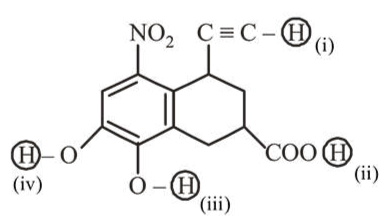# Arrange the following labelled hydrogens in decreasing order of acidity:Question:

Arrange the following labelled hydrogens in decreasing order of acidity:1. (ii) $>$ (i) $>$ (iii) $>$ (iv)

2. (iii) $>$ (ii) $>$ (iv) $>$ (i)

3. (ii) $>$ (iii) $>$ (iv) $>$ (i)

4. (iii) $>$ (ii) $>$ (i) $>$ (iv)

Correct Option: , 3

Solution:

Acidic strength $\propto$ Stability of conjugate base General order of acidic strength is

$\mathrm{R}-\mathrm{COOH}>\mathrm{Ph}-\mathrm{OH}>\mathrm{R}-\mathrm{C} \equiv \mathrm{CH}$

In between (iii) and (iv), (iii) is more acidic due to $-\mathrm{M}$ effect of $-\mathrm{NO}_{2}$.

Thus, decreasing order of acidity is (ii) $>$ (iii) $>$ (iv) $>$ (i).